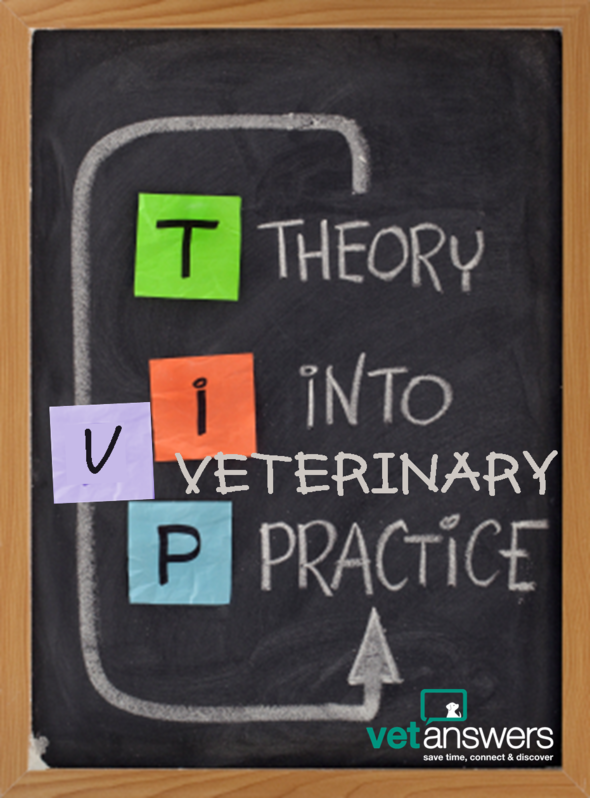Like   Tweet   Pin   +1   inHi Member,

Well, we made it to the last eNews for 2018 - and let me tell you...it's been quite a year (and not in a particularly good way).

But I have also have a lot to be grateful for and 2019 is a new and exciting unknown which I'm looking forward to jumping into, once I've had a wee break. You may not see another eNews until the 1st of February, or maybe the week before - I'll see how things pan out.

In the meanwhile, please look after yourself. It's been a tough year for many people, for many different reasons, and we all need to be kind to each other and most importantly to ourselves. Especially at this time of the year.

Thank you for being a part of Vetanswers and I'm really excited to be growing our Community and introducing all sorts of new tools, resources and information to help make your life as easy as possible in 2019.

Our aim is to help you - save time, connect & discover!table div table+table+table+table div table{width:100%;padding:0}table div table+table+table+table div table img{width:96.23%;padding:0;float:none}table div table+table+table+table div table td{width:100%;padding:0 1.88% 18px}/* styles */Tips for self-care over the Christmas holidays...& every day (Guest Post)

This time of the year can be tough for many people. Looking after ourselves is crucial if we want to maintain a healthy level of wellbeing - physically as well as psychologically. Typically, the Christmas period can be a stressful and lonely time of year for many people, and it’s not uncommon to see a lot of mental health issues rearing their heads around this time.

 table div table+table+table+table+table+table+table div table{width:100%;padding:0}table div table+table+table+table+table+table+table div table img{width:96.23%;padding:0;float:none}table div table+table+table+table+table+table+table div table td{width:100%;padding:0 1.88% 18px}/* styles */Need to talk to someone now?table div table+table+table+table+table+table+table+table+table+table div table{width:100%;padding:0}table div table+table+table+table+table+table+table+table+table+table div table img{width:96.23%;padding:0;float:none}table div table+table+table+table+table+table+table+table+table+table div table td{width:100%;padding:0 1.88% 18px}/* styles */Turn a negative into a positive

By Eric D Garcia (Today's Veterinary Business)

"Knowing how to respond to a client’s less-than-stellar online review can help resolve a conflict effectively and respectfully.”

This post has some great advice if you're not sure how to deal with negative online reviews.

 table div table+table+table+table+table+table+table+table+table+table+table+table+table+table div table{width:100%;padding:0}table div table+table+table+table+table+table+table+table+table+table+table+table+table+table div table img{width:96.23%;padding:0;float:none}table div table+table+table+table+table+table+table+table+table+table+table+table+table+table div table td{width:100%;padding:0 1.88% 18px}/* styles */Love Your Pet Love Your Vet Video #12: What does a positive day look like for you?

In this 3:52 minute video, Dr Anthony Cassimatis, Dr Ophelia Li, Dr Andy Pieris, Dr Scott Campbell & Dr Gerardo Poli discusses what makes for a great day at work.

"The clients that make my day are the ones that are so thankful and grateful for even the little things that I do, who trust me implicitly with their baby - that is the biggest thing for me and they know that their pet is at the top of my list and that's the one thing that we have in common."
- Dr Andy Pieris

"I recognise that we have clients who are rightly emotional at different times and we have clients who are seeking help for their animals out of the blue, and there are clients who have genuine financial limitations, and that's just the world we live in, and all of that's fine. But I guess it's not allowing that stress to affect the communication"
- Dr Scott Campbell

These are great videos to share with your clients and wider community...

 table div table+table+table+table+table+table+table+table+table+table+table+table+table+table+table+table+table+table div table{width:100%;padding:0}table div table+table+table+table+table+table+table+table+table+table+table+table+table+table+table+table+table+table div table img{width:96.23%;padding:0;float:none}table div table+table+table+table+table+table+table+table+table+table+table+table+table+table+table+table+table+table div table td{width:100%;padding:0 1.88% 18px}/* styles */table div table+table+table+table+table+table+table+table+table+table+table+table+table+table+table+table+table+table+table+table+table div table{width:100%;padding:0}table div table+table+table+table+table+table+table+table+table+table+table+table+table+table+table+table+table+table+table+table+table div table img{width:96.23%;padding:0;float:none}table div table+table+table+table+table+table+table+table+table+table+table+table+table+table+table+table+table+table+table+table+table div table td{width:100%;padding:0 1.88% 18px}/* styles */table div table+table+table+table+table+table+table+table+table+table+table+table+table+table+table+table+table+table+table+table+table+table+table+table+table div table{width:100%;padding:0}table div table+table+table+table+table+table+table+table+table+table+table+table+table+table+table+table+table+table+table+table+table+table+table+table+table div table img{width:96.23%;padding:0;float:none}table div table+table+table+table+table+table+table+table+table+table+table+table+table+table+table+table+table+table+table+table+table+table+table+table+table div table td{width:100%;padding:0 1.88% 18px}/* styles */table div table+table+table+table+table+table+table+table+table+table+table+table+table+table+table+table+table+table+table+table+table+table+table+table+table+table+table+table div table{width:100%;padding:0}table div table+table+table+table+table+table+table+table+table+table+table+table+table+table+table+table+table+table+table+table+table+table+table+table+table+table+table+table div table img{width:96.23%;padding:0;float:none}table div table+table+table+table+table+table+table+table+table+table+table+table+table+table+table+table+table+table+table+table+table+table+table+table+table+table+table+table div table td{width:100%;padding:0 1.88% 18px}/* styles */